# Determine the residence time (in hours) for a 25-um particle in the atmosphere with a density of 1,200 kg/m3 at an elevation of 3,500 m. Assume the gravitational acceleration is 9.8 m/s2 and the...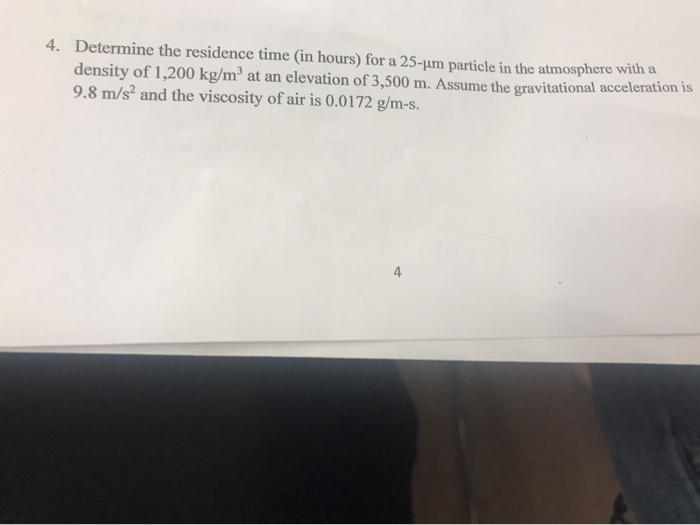Determine the residence time (in hours) for a 25-um particle in the atmosphere with a density of 1,200 kg/m3 at an elevation of 3,500 m. Assume the gravitational acceleration is 9.8 m/s2 and the viscosity of air is 0.0172 g/m-s. 4. 4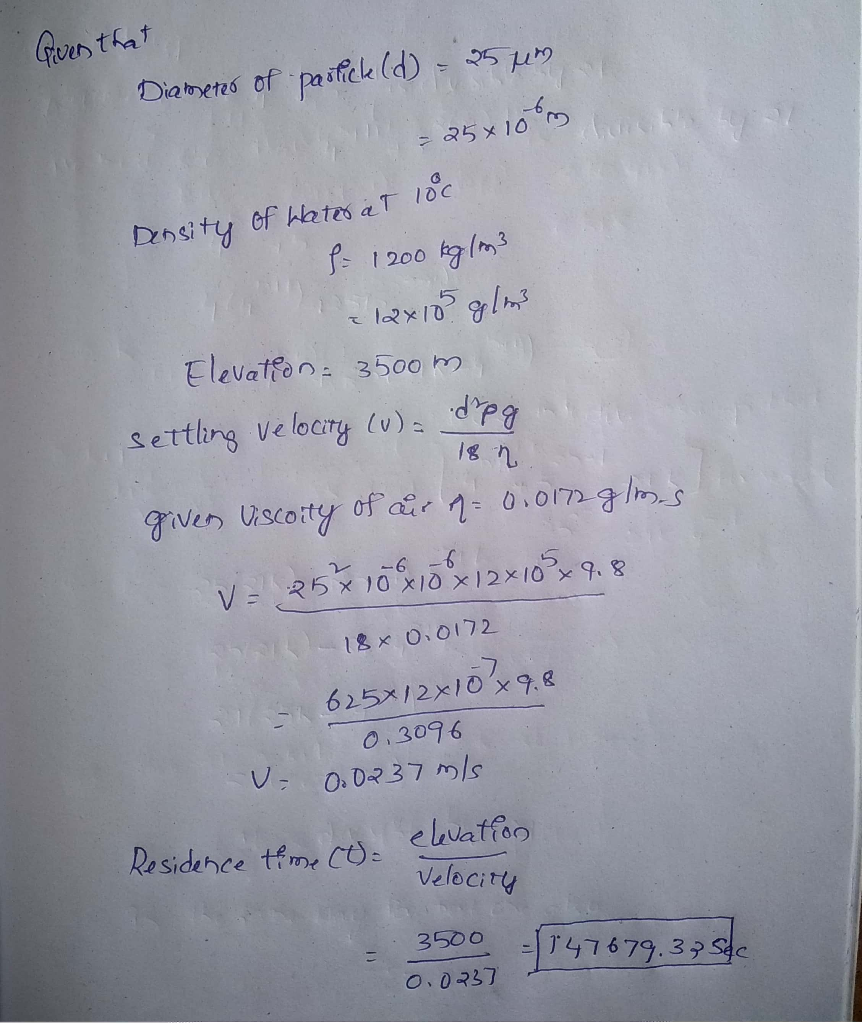#### Earn Coin

Coins can be redeemed for fabulous gifts.

Similar Homework Help Questions
• ### A volcanic plume puts ash into the atmosphere to altitude of 10000m. The characteristic ash particle...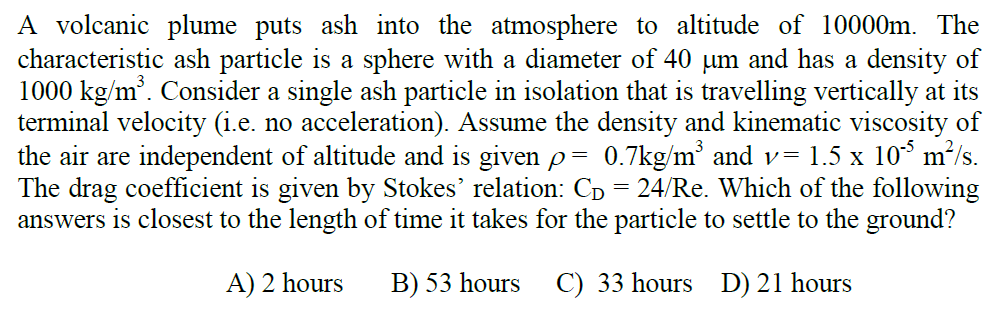A volcanic plume puts ash into the atmosphere to altitude of 10000m. The characteristic ash particle is a sphere with a diameter of 40 um and has a density of 1000 kg/m3. Consider a single ash particle in isolation that is travelling vertically at its terminal velocity (i.e. no acceleration). Assume the density and kinematic viscosity of the air are independent of altitude and is given ,-07kg/m3 and v= 1.5 x 10° m2/s. The drag coefficient is given by Stokes'...

• ### A volcanic plume puts ash into the atmosphere to altitude of 10000m. The characteristic ash particle...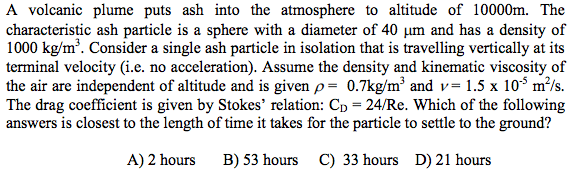A volcanic plume puts ash into the atmosphere to altitude of 10000m. The characteristic ash particle is a sphere with a diameter of 40 mum and has a density of 1000 kg/m^3. Consider a single ash particle in isolation that is travelling vertically at its terminal velocity (i.e. no acceleration). Assume the density and kinematic viscosity of the air are independent of altitude and is given rho = 0.7 kg/m^3 and nu = 1.5 times 10^-5 m^2/s. The drag coefficient...

• ### Question 2 4 pts Question 1lb) If viscous effects are negligible, determine the flow rate Qt (m3/s) at Point 1 in the large pipe if h-5.8 m and V2 66m/s The density of water is 1000 kg/m3. Atm...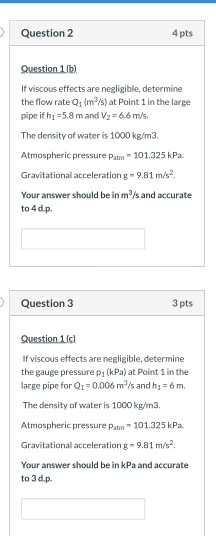Question 2 4 pts Question 1lb) If viscous effects are negligible, determine the flow rate Qt (m3/s) at Point 1 in the large pipe if h-5.8 m and V2 66m/s The density of water is 1000 kg/m3. Atmospheric pressure Patm 101.325 kPa. Gravitational acceleration g- 9.81 m/s Your answer should be in m3/s and accurate to4d.р. Question 3 3 pts If viscous effects are negligible, determine the gauge pressure p1 (kPa) at Point 1 in the large pipe for Q1...

• ### Useful Information: g = 9.8 m/s2 1. A car has a uniform acceleration of 12 m/s²,...

Useful Information: g = 9.8 m/s2 1. A car has a uniform acceleration of 12 m/s², to the right. If it has an initial velocity of 20 m/s (also to the right) what is its final velocity after 4 seconds? 2. A hijacker drops an 80 kg chest of gold from an airplane. Neglecting air resistance, how far does the chest fall in 6 seconds? 3. The distance between two objects which exert a gravitational force on each other is...

• ### (1) An object moves with constant acceleration 3.26 m/s and over a time interval reaches a...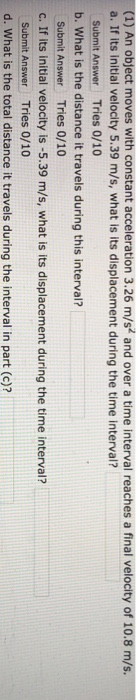(1) An object moves with constant acceleration 3.26 m/s and over a time interval reaches a final velocity of 10.8 m/s. a. If its initial velocity 5.39 m/s, what is its displacement during the time interval? Submit Answer Tries 0/10 b. What is the distance it travels during this interval? Submit Answer Tries 0/10 c. If its initial velocity is -5.39 m/s, what is its displacement during the time interval? Submit Answer Tries 0/10 d. What is the total distance...

• ### In which of the following units is acceleration expressed

1. In which of the following units is acceleration expressed?*A. NewtonsB. Foot-poundsC. KilogramsD. Meters per second squared2. A stone falls from a ledge and takes 16 seconds to hit theground. The stone has an original velocity of 0 m/s. How tall isthe ledge?A. 1,254.4 meters *C. 156.8 metersB. 313.6 meters D. 78.4 meters3. A tank with a flat bottom is filled with water to a height of 3.5 meters. What is the pressureat any point at the bottom of the...